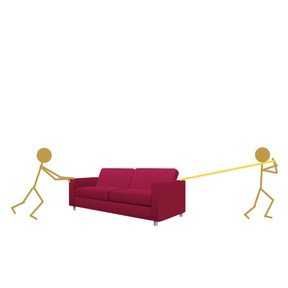Introduction to forces

# Introduction to forces

I can describe how forces can add to or cancel out one another.8,000 schools use Gynzy92,000 teachers use Gynzy1,600,000 students use Gynzy

## General

This lesson is a more in-depth investigation of balanced and unbalanced forces. Students will learn the formal definition of a force (including its si unit Newtons). Students will learn how to add and subtract forces to find the net force. They will learn how balanced and unbalanced forces act upon objects at rest and objects in motion.

NGSS: MS-PS2-2

## Learning objective

Students will be able to describe how forces can add to or cancel out one another.

## Introduction

Students will do a think, pair, share about tug-of-war.

## Instruction

Explain that a force is a push or pull that acts on an object. Students will look at a picture of a girl on a skateboard and discuss which way they think the girl will move. They will look at a scenario where there's more than one force acting on an object in the same direction.

Explain that net force is the combination of individual forces acting on an object. If the forces are pointed in the same direction, the net force is the sum of the forces. If the forces are pointed in opposite direction, the net force is the difference. They will look at a scenario where two forces are acting in opposite directions. Next, discuss unbalanced forces.

## Quiz

Students will respond to ten true/false and multiple-choice questions.

## Closing

Students will complete an exit slip about the lesson.

### The online teaching platform for interactive whiteboards and displays in schools

• Save time building lessons

• Manage the classroom more efficiently

• Increase student engagement